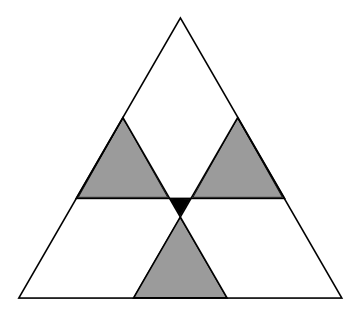You may also likeConsecutive Numbers

An investigation involving adding and subtracting sets of consecutive numbers. Lots to find out, lots to explore.Calendar Capers

Choose any three by three square of dates on a calendar page...Days and Dates

Investigate how you can work out what day of the week your birthday will be on next year, and the year after...

Age 11 to 14 ShortChallenge LevelThe diagram shows a large equilateral triangle divided by three straight lines into seven regions. The three grey regions are equilateral triangles with sides of length $5\;\mathrm{cm}$ and the central black region is an equilateral triangle with sides of length $2\;\mathrm{cm}$.

What is the side length of the original large triangle?

If you liked this problem, here is an NRICH task that challenges you to use similar mathematical ideas.

This problem is taken from the UKMT Mathematical Challenges.
You can find more short problems, arranged by curriculum topic, in our short problems collection.